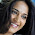### Infix to Prefix conversion using Stack

This post is about conversion of Infix expression to Prefix conversion. For this conversion we take help of stack data structure, we need to push and pop the operators in and out of the stack.

Infix expressions are the expressions that we normally use,eg. 5+6-7; a+b*c etc. Prefix expressions are the expressions in which the 2 operands are preceded by the operator eg. -+567 , +a*bc etc.

This method is very similar to the method that we used to convert Infix to Postfix but the only difference is that here we need to reverse the input string before conversion and then reverse the final output string before displaying it.

NOTE: This changes one thing that is instead of encountering the opening bracket we now first encounter the closing bracket and we make changes accordingly in our code.

So, to convert an infix expression to a prefix expression we follow the below steps
(we have 2 string, 1st is the input infix expression string 2nd is the output string which is empty initially)

1. We first reverse the input infix expression.
2. We then start by going through the characters of the infix expression one by one.
3. If we come across an operand we simply copy it to the Prefix output string.
4. If we come across any closing parenthesis we push it on the stack.
5. If we come across any opening parenthesis we pop the stack till we find the corresponding closing parenthesis.
6. If we come across an operator then we have 2 cases based on the precedence of the operators
1. If the current operator has precedence higher than or equal to the stack top then we push the current operator on the stack.
2. If the current operator has precedence less than the stack top then we pop the operator at the top and put it to the output string and then check the above condition again with the new stack top.
7. Finally, we reverse the prefix output string.

To see Simple Stack implementation program, click Here.

### Sample input and output to check the program

You might also be interested in

Infix to Postfix Conversion
Insertion Sort
Selection sort
Shell Sort
Hashing with Linear Probing
Double Hashing
4 methods to swap 2 numbers

1.I like your blog, I read this blog please update more content on python, further check it once at python online training

### Popular posts from this blog

Hashing is a technique used for storing , searching and removing elements in almost constant time. Hashing is done with help of a hash function that generates index for a given input, then this index can be used to search the elements, store an element, or remove that element from that index.

A hash function is a function that is used to map the data elements to their position in the data structure used. For example if we use an array to store the integer elements then the hash function will generate position for each element so that searching, storing and removing operation on the array can be done in constant time that is independent of the number of elements in the array. For better look at the example below.

now we face a problem if for 2 numbers same position is generated example consider elements 1 and 14

1 % 13 = 1

14 % 13 = 1

so when we get 1 we store it at the first position, but when we get 14 we see that the position 1 is already taken, this is a case of collision.

Inorder…